IMO Shortlist 2013 problem N7

Kvaliteta:
Avg: 0,0
Težina:
Avg: 9,0
Let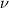$\nu$ be an irrational positive number, and let$m$ be a positive integer. A pair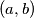$(a, b)$ of positive integer is called good if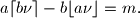A good pair$(a, b)$ is called excellent if neither of the pairs$(a - b, b)$ and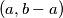$(a, b - a)$ is good. (As usual, by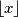$\lfloor x \rfloor$ and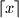$\lceil x \rceil$ we denote the integer numbers such that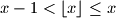$x - 1 < \lfloor x \rfloor \leq x$ and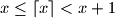$x \leq \lceil x \rceil < x + 1$.)

Prove that the number of excellent pairs is equal to the sum of the positive divisors of$m$.
Izvor: U.S.A.